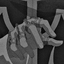Ask your own question, for FREE!
Mathematicsjhonyy9:

few days ago somebody asked me this math problem : inside a square there is a circle ,suppose the perimeter of the square is equal with the circumference of the circle - maybe this true ? and why not can have the same ,equal them areas too ?jhonyy9:

|dw:1614420550505:dw| the circumference of the circle : $C_o = 2pir =6,28r$ the perimeter of the square : P_ABCD = 4*2r = 8r C_o not can be equal P_ABCD - about these areas : area of the circle : $A_o = \pi*r^2 = 3,14*r^2$ area of the square : $A.\square = 2r*2r = 4r^2$jhonyy9:

$$\color{#0cbb34}{\text{Originally Posted by}}$$ @jhonyy9 Created with RaphaëlOrABCDReply Using Drawing the circumference of the circle : $C_o = 2pir =6,28r$ the perimeter of the square : $P_\square = 4*2r = 8r$ $C_o \not = P_\square$ - about these areas : area of the circle : $A_o = \pi*r^2 = 3,14*r^2$ area of the square : $A.\square = 2r*2r = 4r^2$ $$\color{#0cbb34}{\text{End of Quote}}$$jhonyy9:

the second case |dw:1614422034533:dw|jhonyy9:

in this second case we get $AB = r \sqrt{2}$ so the perimeter of the circle will be $Co = 2\pi*r = 6,28r$ the perimeter of the square : $P_\square = 4r \sqrt{2} = 4,56r$ - about areas - area of the circle $Ao = \pi*r^2 = 3,14*r^2$ - area of the square $A_\square = r \sqrt{2} *r \sqrt{2} = 2r^2$ opinions please ??? ty.sxdsouls:

Im not sure what this is because I havent learned this YET. But by seeing this its good, great job (:jhonyy9:

$$\color{#0cbb34}{\text{Originally Posted by}}$$ @sxdsouls Im not sure what this is because I havent learned this YET. But by seeing this its good, great job (: $$\color{#0cbb34}{\text{End of Quote}}$$ ok ty so muchsxdsouls:

No problem I enjoy watching you do math for some reason :)jhonyy9:

$$\color{#0cbb34}{\text{Originally Posted by}}$$ @sxdsouls No problem I enjoy watching you do math for some reason :) $$\color{#0cbb34}{\text{End of Quote}}$$ okMxxnLight:

Look's great, thank's jhonny! (:Downpour17:

Great job my fellow European QC user!AngryPomeranian:

Good Jobdarkknight:

long story short, ye if a circle is inscribed in a square, half of the squares length will be equal to the radius of the circle be definition so for perimeter of the circle $2\pi*r$ whereas perimeter of the square $2*r*4$ now 8r only equals 2pi*r when r =0, which theoretically is not a shape (because if r = 0 there is no shape). Same stuff with the area We do 2r (for the full side length) and square this $4r^2$ is area of square area of circle is $\pi*r^2$ $4r^2=\pi*r^2$ $4\neq \pi$darkknight:

So you just proved a circle inscribed in a square cant have the same circum or area, also if you look at the pictures you can see clearly that the area can not be the same, there are parts left out, whereas the circum can only be the same when r= 0 (so basically never)darkknight:

In other words, yes I think you did this rightb1az3:

Can't find your answer? Make a FREE account and ask your own questions, OR help others and earn volunteer hours!

Join our real-time social learning platform and learn together with your friends!
Latest Questionskatelyn865: whats the coolst thing you evey seen
17 seconds ago 5 Replies 1 Medalvybez: help ;o
4 minutes ago 19 Replies 1 Medalkristiannnnnt: What's your average WPM?
21 minutes ago 0 Replies 0 MedalsSingleGirl123: What inequality does the number line graph represent?
10 minutes ago 8 Replies 0 Medalskristiannnnnt: RATE
17 minutes ago 13 Replies 1 MedalForeverangel: What does the number next to the element name indicate?
46 minutes ago 5 Replies 0 Medalsfernandez06: HELP Point D is the center of the inscribed circle of ABC. Which step can you use to draw the inscribed circle of ABC? A.
54 minutes ago 0 Replies 0 Medalsfernandez06: Point D is the center of the inscribed circle of ABC. Which step can you use to draw the inscribed circle of ABC? A.
56 minutes ago 0 Replies 0 Medalskristiannnnnt: help
57 minutes ago 1 Reply 0 MedalsSaltTheLoser: Help
50 minutes ago 21 Replies 2 Medals
Can't find your answer? Make a FREE account and ask your own questions, OR help others and earn volunteer hours!

Join our real-time social learning platform and learn together with your friends!